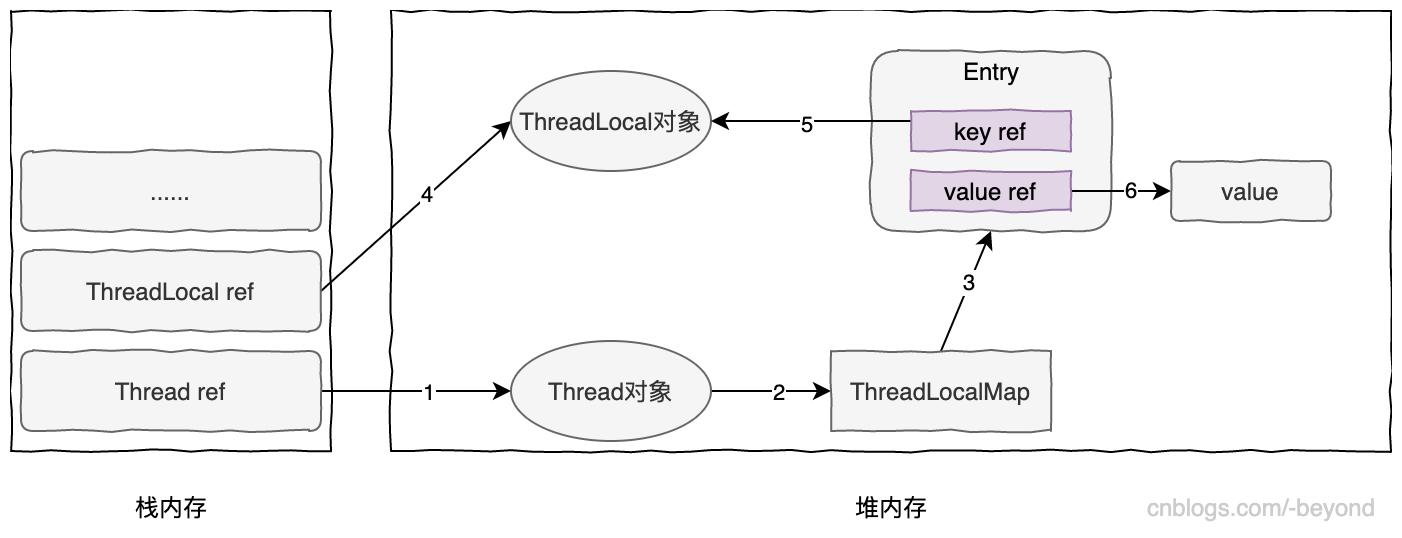2、变量不是线程安全的，然而我们还不希望通过同步的方式来实现，因为本身采用同步锁就影响性能。

`````` private static final ThreadLocal<SimpleDateFormat> dateFormat = new ThreadLocal<SimpleDateFormat>(){
@Override
protected SimpleDateFormat initialValue(){
return new SimpleDateFormat("dd/MM/yyyy");
}
};
``````

``````  static class Entry extends WeakReference<ThreadLocal<?>> {
/** The value associated with this ThreadLocal. */
Object value;

super(k);
value = v;
}
}````````````public void execute() {

doOne();
doTwo();

}

=public void doOne() {
tl.set(2L);
}
public void doTwo() {
tl.set(99);
}``````

``````               if (k == null) {
e.value = null;
tab[i] = null;
size--;
}``````

``````public class WeakReference<T> extends Reference<T> {

/**
* Creates a new weak reference that refers to the given object.  The new
* reference is not registered with any queue.
*
* @param referent object the new weak reference will refer to
*/
public WeakReference(T referent) {
super(referent);
}

/**
* Creates a new weak reference that refers to the given object and is
* registered with the given queue.
*
* @param referent object the new weak reference will refer to
* @param q the queue with which the reference is to be registered,
*          or <tt>null</tt> if registration is not required
*/
public WeakReference(T referent, ReferenceQueue<? super T> q) {
super(referent, q);
}

}
``````

``````String s= new String("dd");
WeakReference<String> weakR = new WeakReference<String>(s);

weakR.get()

s = null
``````

Tomcat中即使用了WeakHashMap，其设置了两级本地缓存，第一层是比较热点的缓存数据，第二层则是相对不是很热点的数据。第二层的数据在只有弱引用时就会被回收。

``````public final class ConcurrentCache<K, V> {
private final int size;
private final Map<K, V> eden;
private final Map<K, V> longterm;

public ConcurrentCache(int size) {
this.size = size;
this.eden = new ConcurrentHashMap(size);
this.longterm = new WeakHashMap(size);
}

public V get(K k) {
V v = this.eden.get(k);
if (v == null) {
synchronized(this.longterm) {
v = this.longterm.get(k);
}

if (v != null) {
this.eden.put(k, v);
}
}

return v;
}

public void put(K k, V v) {
if (this.eden.size() >= this.size) {
synchronized(this.longterm) {
this.longterm.putAll(this.eden);
}

this.eden.clear();
}

this.eden.put(k, v);
}
}``````

--------EOF---------